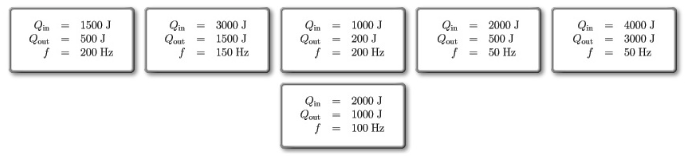# Problem: Rank these engines on the basis of their designed power output. Rank from largest to smallest. To rank items as equivalent, overlap them.

###### FREE Expert Solution

Power:

$\overline{){\mathbf{P}}{\mathbf{=}}\frac{\mathbf{W}}{\mathbf{t}}}$

Where P is power, W is work and t is time.

Work:

$\overline{){\mathbf{W}}{\mathbf{=}}{{\mathbf{Q}}}_{\mathbf{i}\mathbf{n}}{\mathbf{-}}{{\mathbf{Q}}}_{\mathbf{o}\mathbf{u}\mathbf{t}}}$

Frequency:

$\overline{){\mathbf{f}}{\mathbf{=}}\frac{\mathbf{1}}{\mathbf{T}}}$

where T is periodic time.

(1/t) = f

P = W/t = (Qin - Qout)•f

88% (125 ratings)###### Problem Details

Rank these engines on the basis of their designed power output. Rank from largest to smallest. To rank items as equivalent, overlap them.